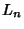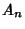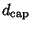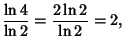## Tetrix

The 3-D analog of the Sierpinski Sieve illustrated above, also called the Sierpinski Sponge or Sierpinski Tetrahedron. Letbe the number of tetrahedra,the length of a side, andthe fractional Volume of tetrahedra after theth iteration. Then(1)(2)(3)

The Capacity Dimension is therefore(4)

so the tetrix has an Integral Capacity Dimension (albeit one less than the Dimension of the 3-D Tetrahedra from which it is built), despite the fact that it is a Fractal.

The following illustration demonstrates how this counterintuitive fact can be true by showing three stages of the rotation of a tetrix, viewed along one of its edges. In the last frame, the tetrix looks'' like the 2-D Plane.Dickau, R. M. Sierpinski Tetrahedron.'' http://forum.swarthmore.edu/advanced/robertd/tetrahedron.html.
Eppstein, D. Sierpinski Tetrahedra and Other Fractal Sponges.'' http://www.ics.uci.edu/~eppstein/junkyard/sierpinski.html.